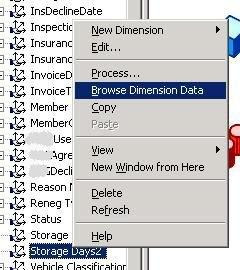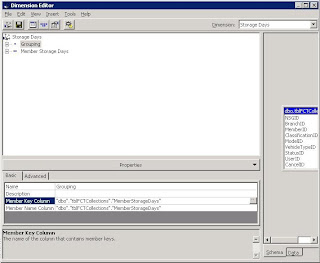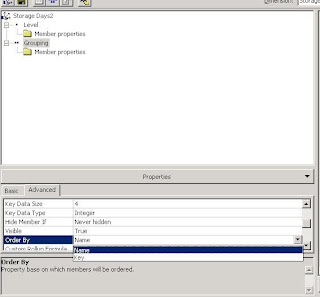## Thursday, 21 September 2006

### Analysis Services - Grouping numbers in a higher level

In this blog I am going to explain how to create a new level in a dimension - effectively grouping items together.
Firstly lets have a look at the dimension and show you what data is there. This is done by right clicking on the dimension within Analysis Manager, then clicking on Browse dimension dataThe results of this are shown belowSo the first thing to do is add the new level. Close the open window and right click again on the dimension and select edit. Then right click on the current level and select new level (shown below)Now select the same member key you had beforeNow for the important bit. If you click on the level and look at its properties you can see it has a value for member key column and member name column shown below.What this basically means is group all the keys by this value - in this case everything with the same number of storage days will be grouped together. What we want to do is group the numbers by 25. So what we do is divide the number by 25 - because sql handles this as an int, and rounds down you get the following:-
1/25 = 0
24/25 = 0
26/25 = 1
50/25 = 2
And so on. So it is this that does the grouping. For the naming we need to use SQLs rounding again. What we are after is the following string
x - y
Where x is the lowest number in the group, and y is the biggest.
This is the statement we use:-
cast((("dbo"."tblFCTCollections"."MemberStorageDays")/25) *25 as varchar) + ' - ' + cast((("dbo"."tblFCTCollections"."MemberStorageDays")/25) *25 + 24 as varchar)
The first value divides the current number by 25, then times it by 25 - this produces:-
1/25 * 25 = 0
24/25 * 25 = 0
26/25 * 25 = 25
50/25 * 25 = 50
we then cast this to a string and add " - ". We then take the previous number and simply add 24
1/25 * 25 + 24 = 24
24/25 * 25 + 24 = 24
26/25 * 25 + 24 = 49
50/25 * 25 + 24 = 74
Simple! This is shown below.What we finally need to do is say that member names must be unique - this stops us having a group for every value. This is shown belowAnd if we take a look at the data we can see it correctly groupedand if we expand a group you can see the items beneath it:As you can see it is completely unordered - ordering it correctly is easy though, simply go back into the editing menu and change the ordering from name to key, shown belowAs you can see this fixes the ordering and all is well with the world once more!Thanks for reading this! If you know of an easier way to do this then let me know!